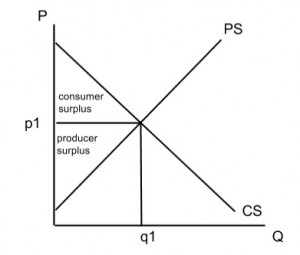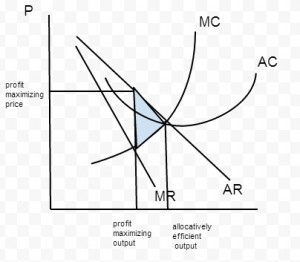## (a) Explain how welfare loss may result from monopoly power.

M13/3/ECONO/HP1/ENG/TZ1/XX

– Explain characteristics of monopoly (single seller, price setter, unique product, BTE are high)

– Explain that welfare loss arises because the firms fail to allocate resources efficiently (they are not allocating at the optimal output which maximizes producer/consumer welfare) & are productive inefficient

– Explain how welfare is maximized (welfare is maximized at socially optimal output at Q where DD=SS, or AR=MC). this is where the allocation is said to be pareto optimal- where nobody can be made better off without anyone made worse off*this is the diagram that shows welfare being maximized, it is not required and purely for your understanding of welfare being maximized or allocative efficiency achieved

– Because M is a price setter, it is able to determine its own price at MC=MR(which is the profit max level of output), assuming that maximize profits– Therefore it earns more profit by producing less than the socially optimal output at Q1, it restricts output so that it can charge a higher price to max profit

– Also, the firm is productive inefficient because it fails to produce at the min AC; ie it is not maximizing the use of resources. It usually produces to the left of min AC, suggesting inability to maximize capacity

– Hence, there is under-allocation in this market, resulting in a loss of welfare represented by the red triangle (DWL)